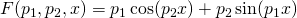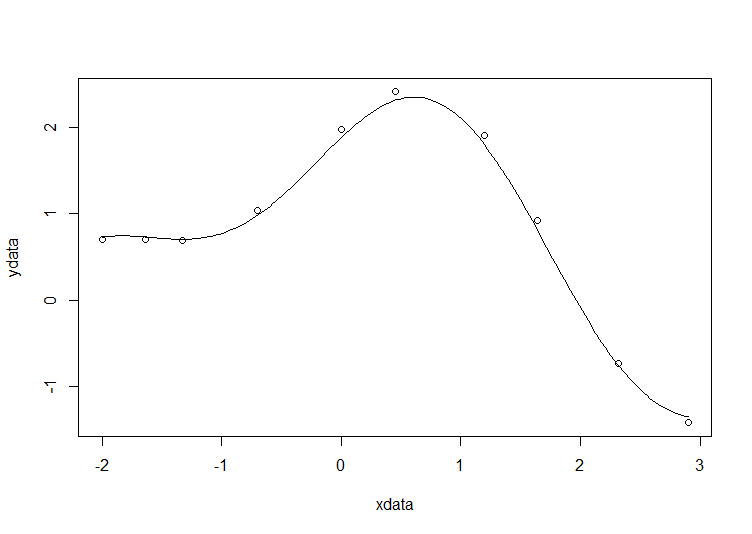Simple nonlinear least squares curve fitting in R

December 6th, 2013 | Categories: math software, R | Tags:

The R code used for this example comes from Barry Rowlingson, so huge thanks to him.

A question I get asked a lot is ‘How can I do nonlinear least squares curve fitting in X?’ where X might be MATLAB, Mathematica or a whole host of alternatives.  Since this is such a common query, I thought I’d write up how to do it for a very simple problem in several systems that I’m interested in

This is the R version. For other versions,see the list below

The problem

xdata = -2,-1.64,-1.33,-0.7,0,0.45,1.2,1.64,2.32,2.9
ydata = 0.699369,0.700462,0.695354,1.03905,1.97389,2.41143,1.91091,0.919576,-0.730975,-1.42001

and you’d like to fit the functionusing nonlinear least squares.  You’re starting guesses for the parameters are p1=1 and P2=0.2

For now, we are primarily interested in the following results:

• The fit parameters
• Sum of squared residuals
• Parameter confidence intervals

Future updates of these posts will show how to get other results. Let me know what you are most interested in.

Solution in R

# construct the data vectors using c()
xdata = c(-2,-1.64,-1.33,-0.7,0,0.45,1.2,1.64,2.32,2.9)
ydata = c(0.699369,0.700462,0.695354,1.03905,1.97389,2.41143,1.91091,0.919576,-0.730975,-1.42001)

# look at it
plot(xdata,ydata)

# some starting values
p1 = 1
p2 = 0.2

# do the fit
fit = nls(ydata ~ p1*cos(p2*xdata) + p2*sin(p1*xdata), start=list(p1=p1,p2=p2))

# summarise
summary(fit)

This gives

Formula: ydata ~ p1 * cos(p2 * xdata) + p2 * sin(p1 * xdata)

Parameters:
Estimate Std. Error t value Pr(>|t|)
p1 1.881851   0.027430   68.61 2.27e-12 ***
p2 0.700230   0.009153   76.51 9.50e-13 ***
---
Signif. codes:  0 ‘***’ 0.001 ‘**’ 0.01 ‘*’ 0.05 ‘.’ 0.1 ‘ ’ 1

Residual standard error: 0.08202 on 8 degrees of freedom

Number of iterations to convergence: 7
Achieved convergence tolerance: 2.189e-06

Draw the fit on the plot by getting the prediction from the fit at 200 x-coordinates across the range of xdata

new = data.frame(xdata = seq(min(xdata),max(xdata),len=200))
lines(new\$xdata,predict(fit,newdata=new))Getting the sum of squared residuals is easy enough:

sum(resid(fit)^2)

Which gives

 0.0538127

Finally, lets get the parameter confidence intervals.

confint(fit)

Which gives

Waiting for profiling to be done...
2.5%     97.5%
p1 1.8206081 1.9442365
p2 0.6794193 0.7209843
1. Of course, I had to try this in Euler. Find the solution on

http://observations.rene-grothmann.de/non-linear-fit-in-euler-math-toolbox/

Yours, R.G.

2. You say “This is the MATLAB version. For other versions,see the list below” – Should be “This is the R version. For other versions,see the list below”

3. Thanks…a copy and paste error. Fixed now :)

4. Thanks R.G. — looks good

5. Fun exercise. I posted a solution to the JMP blog using the JMP scripting language.

http://blogs.sas.com/content/jmp/2013/12/18/simple-nonlinear-least-squares-curve-fitting-in-jmp/

6. Thanks , very good,Now, i am starting to know the nls and curve fitting a little bit,

7. Thanks for this tutorial, very helpful!

8. I would like to fit the nonlinear equation using r for the data set, how do I get it right

Day %Cum aggregates
0 0
15 0
45 0
75 4.5
105 19.7
135 39.5
165 59.2
195 77.1
225 93.6
255 98.7
285 100
315 100

Fit1 equation
100*(1+((p1*x)^p2)^-(1-1/p2))^-p3)

x=day, y=%Cumulative aggregate
where p1,p2,p3 are parameters (initial p=0.01, p2=10, p3=0.5

Fit2 equation
100*(1+((p1*x)^p2)^-(1-1/p2))^-0.5)

9. x=c(0,15,45,75,105,135,165,195,225,255,285,315)
y=c(0,0,0,4.5,19.7,39.5,59.2,77.1,93.6,98.7,100,100)